Rotational Symmetry

Chapter 14 Class 7 Symmetry
Concept wise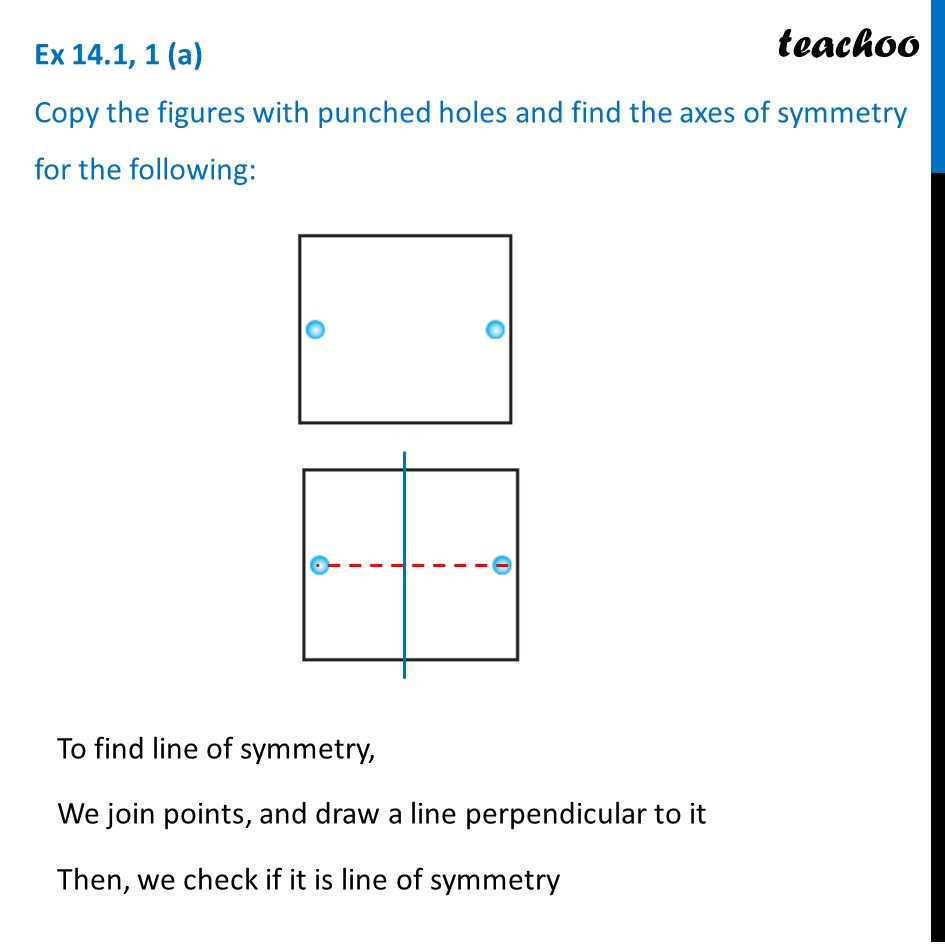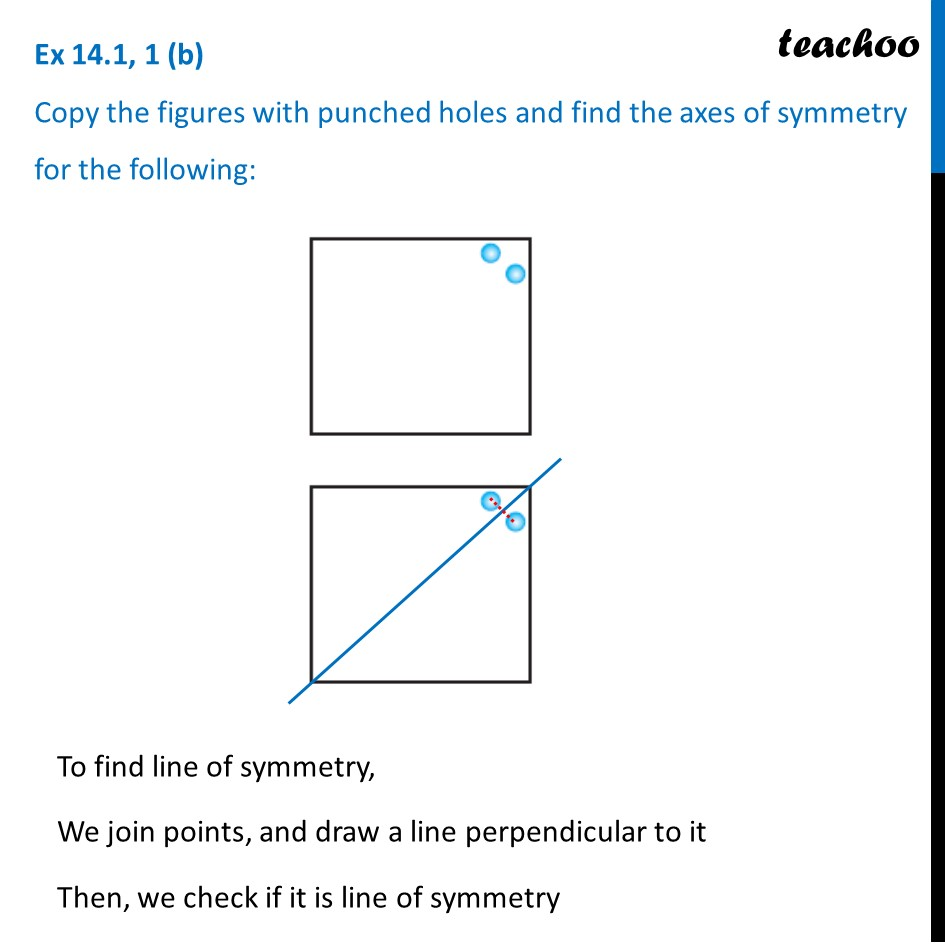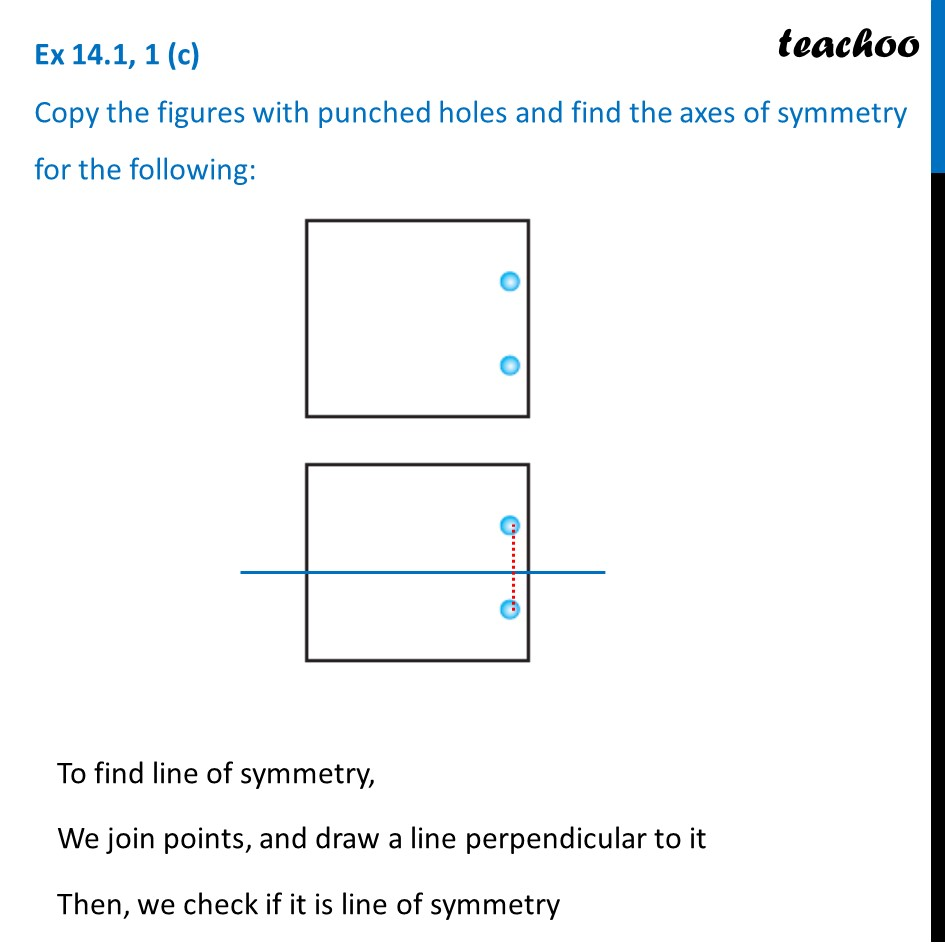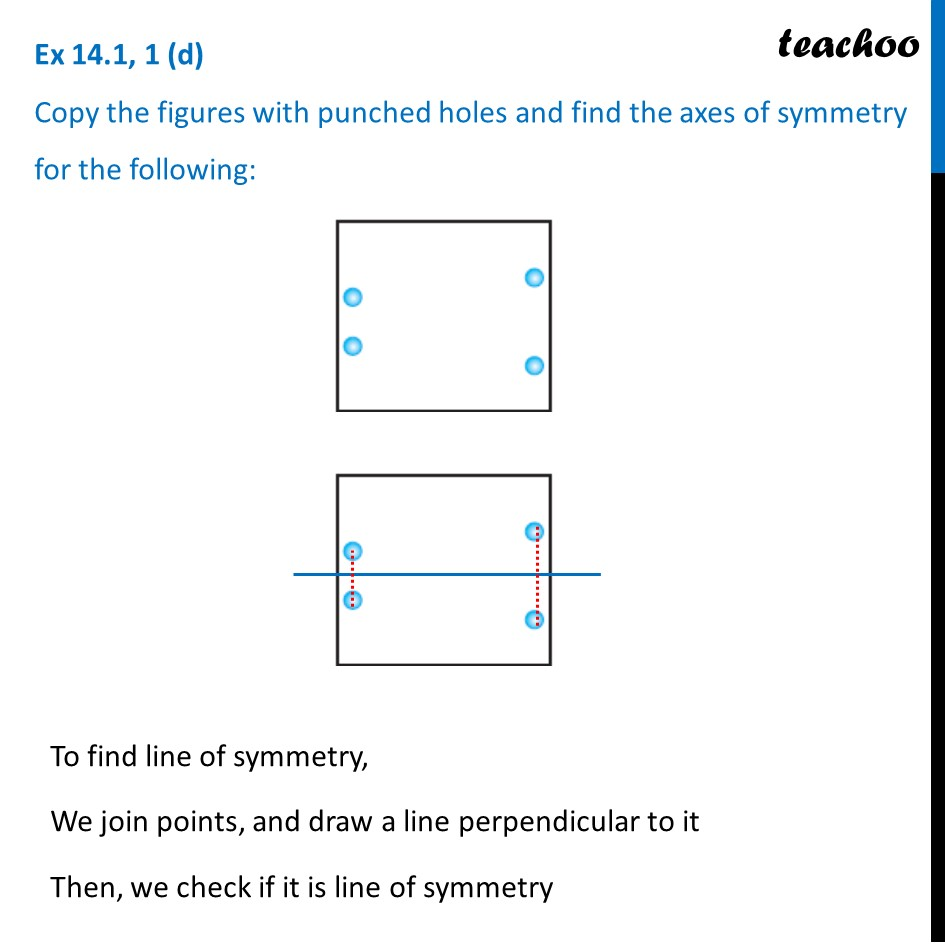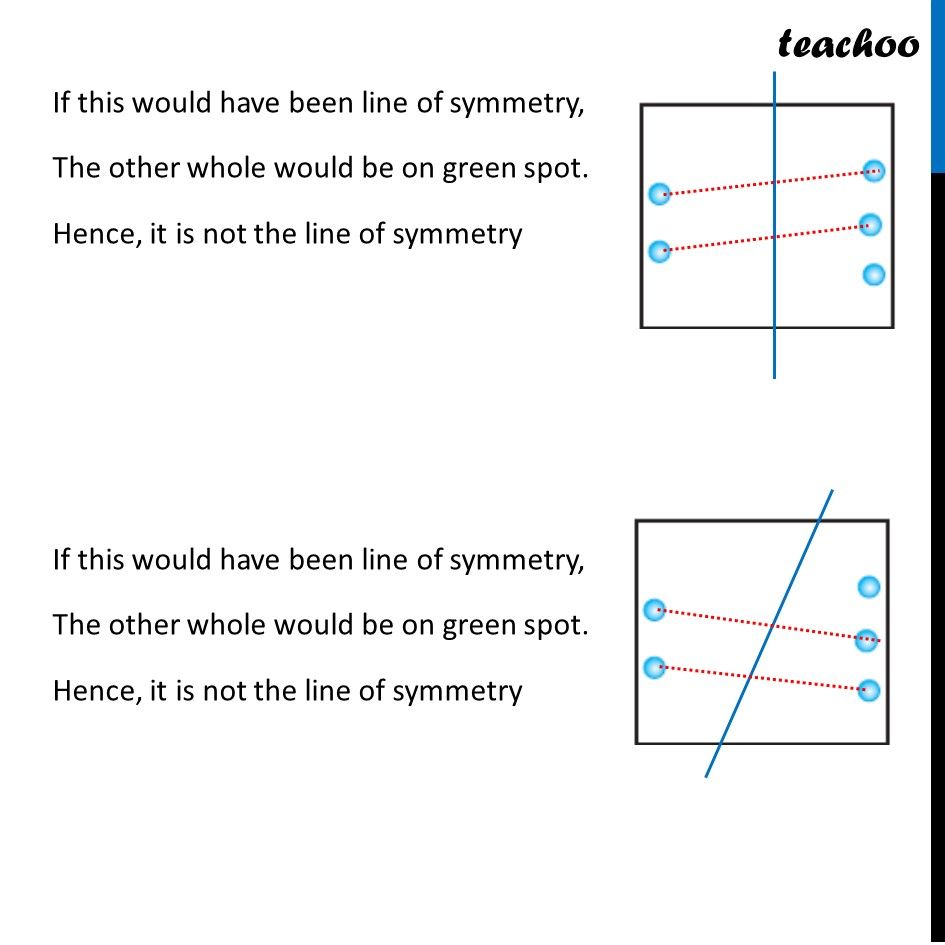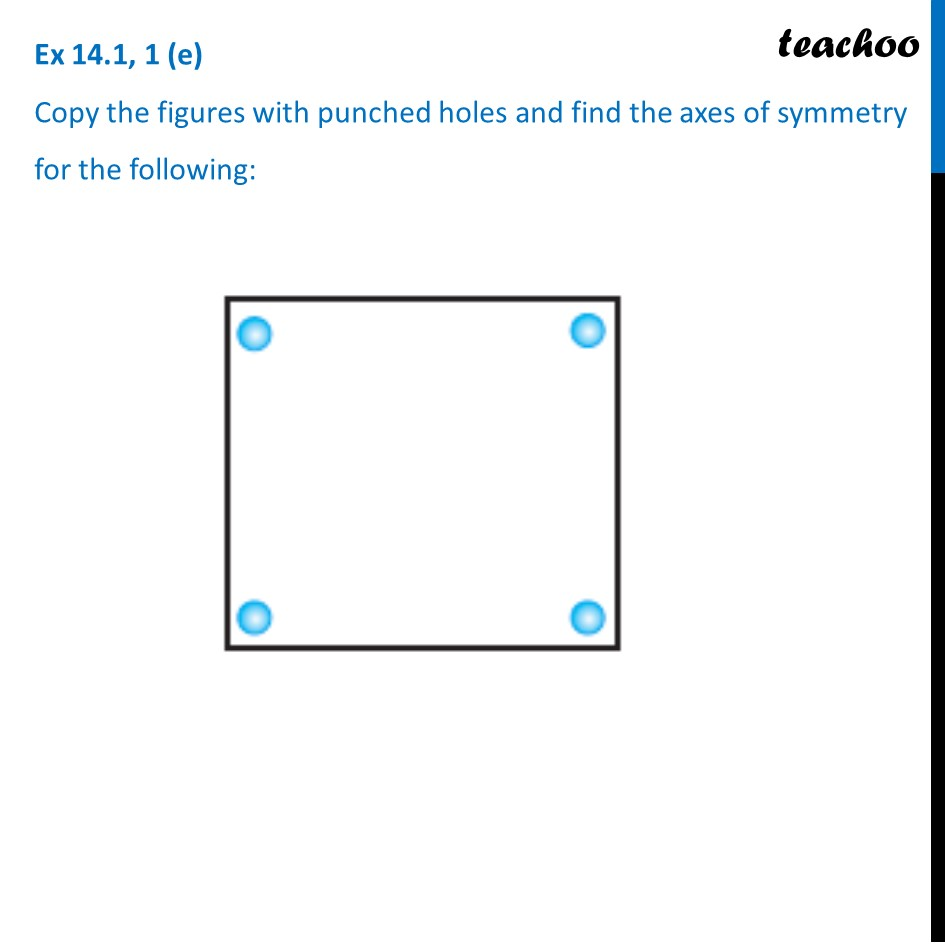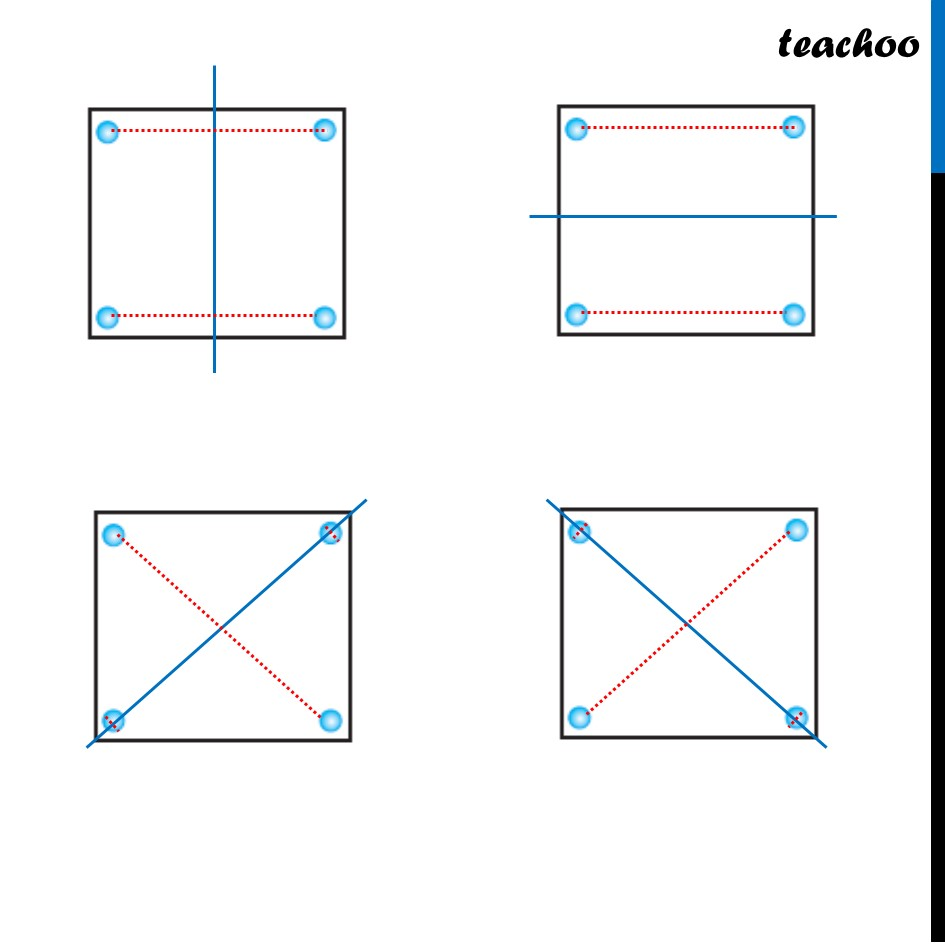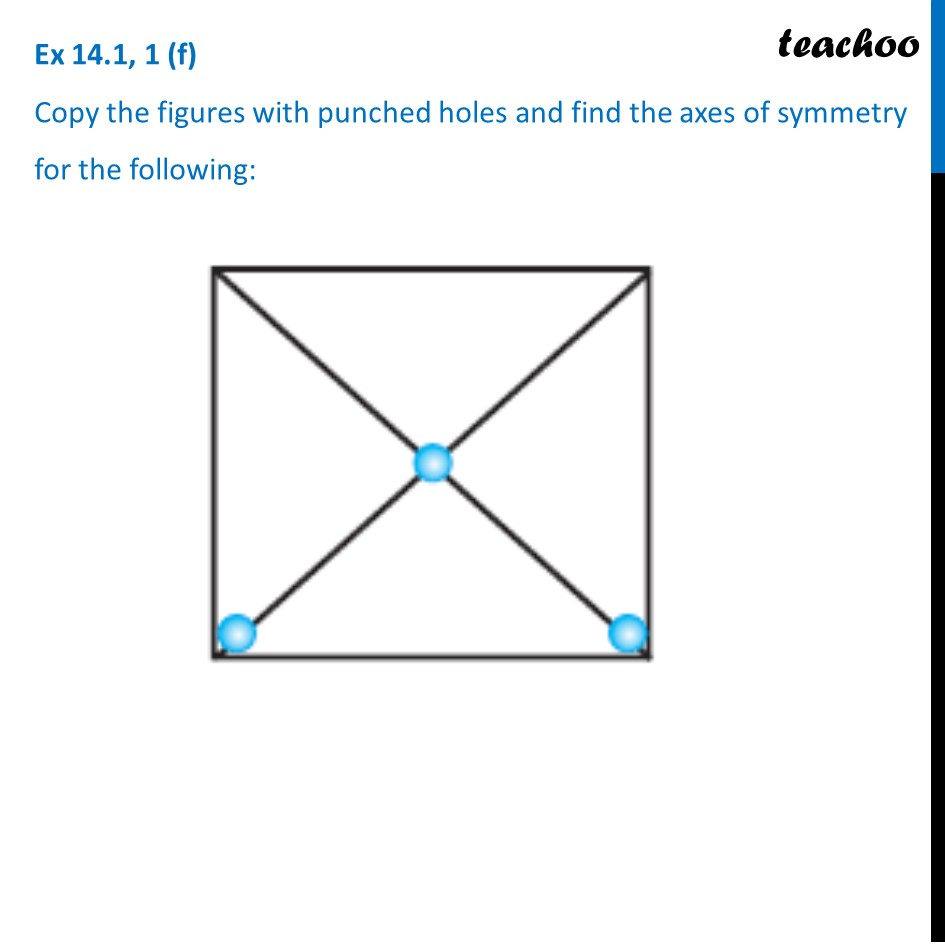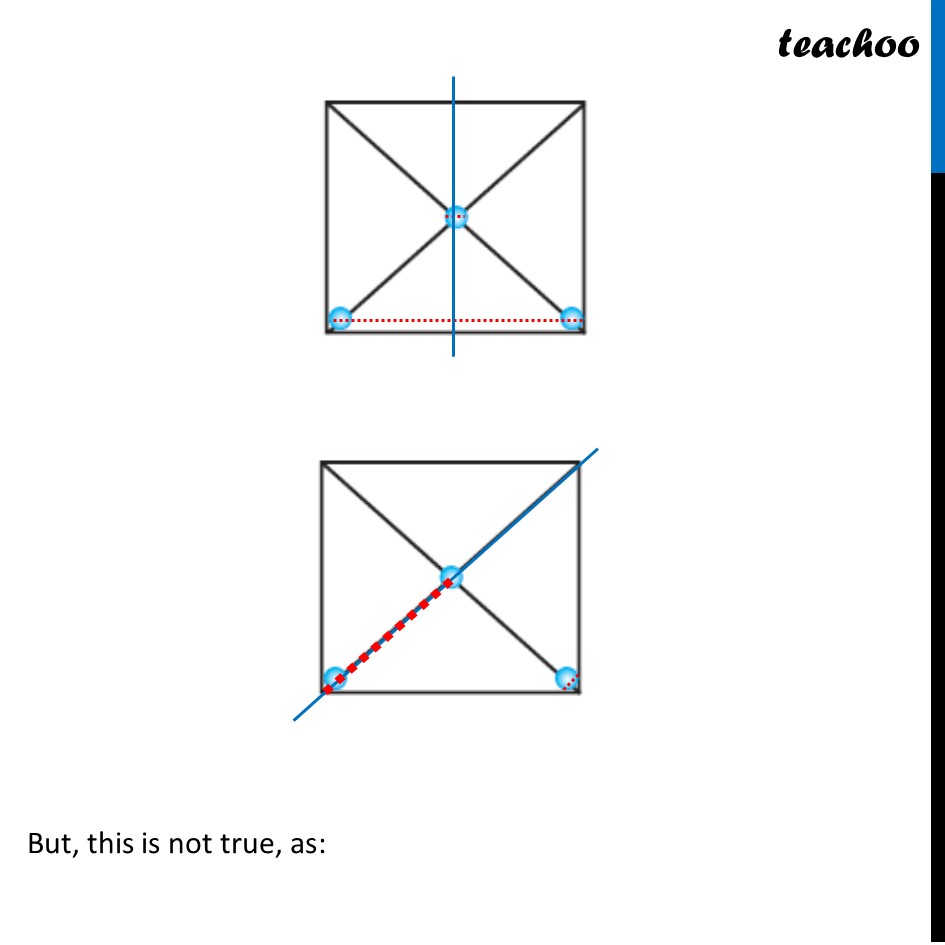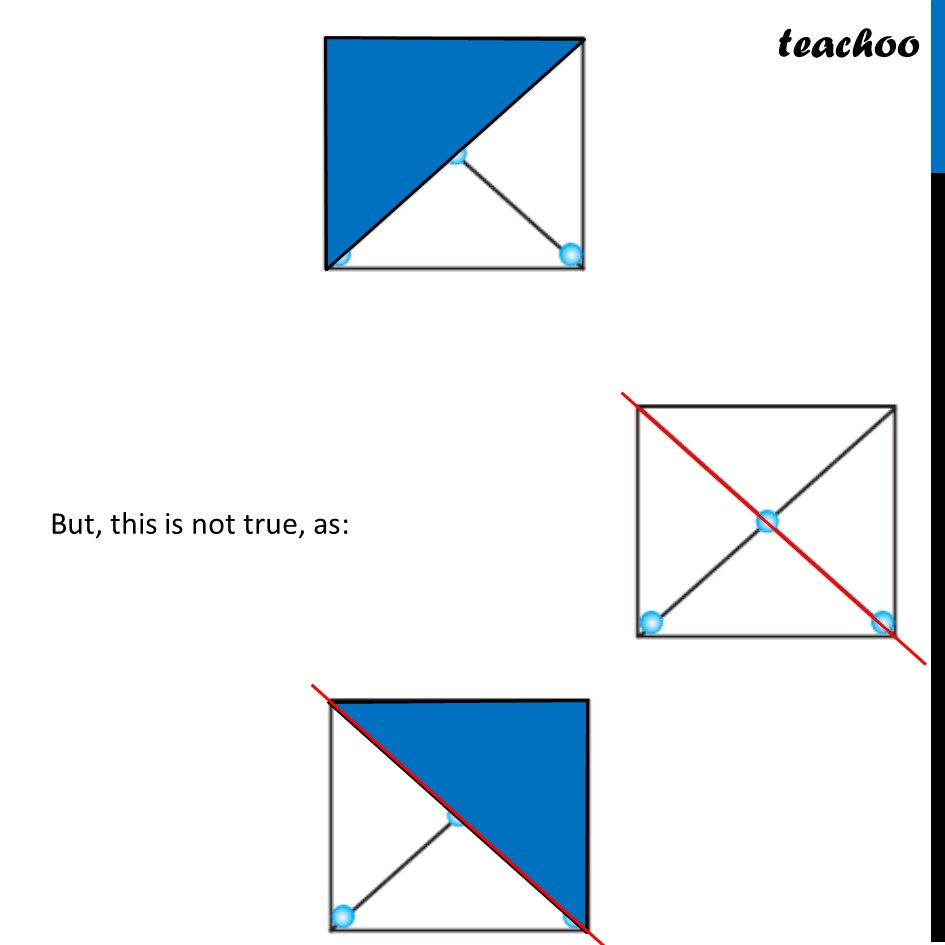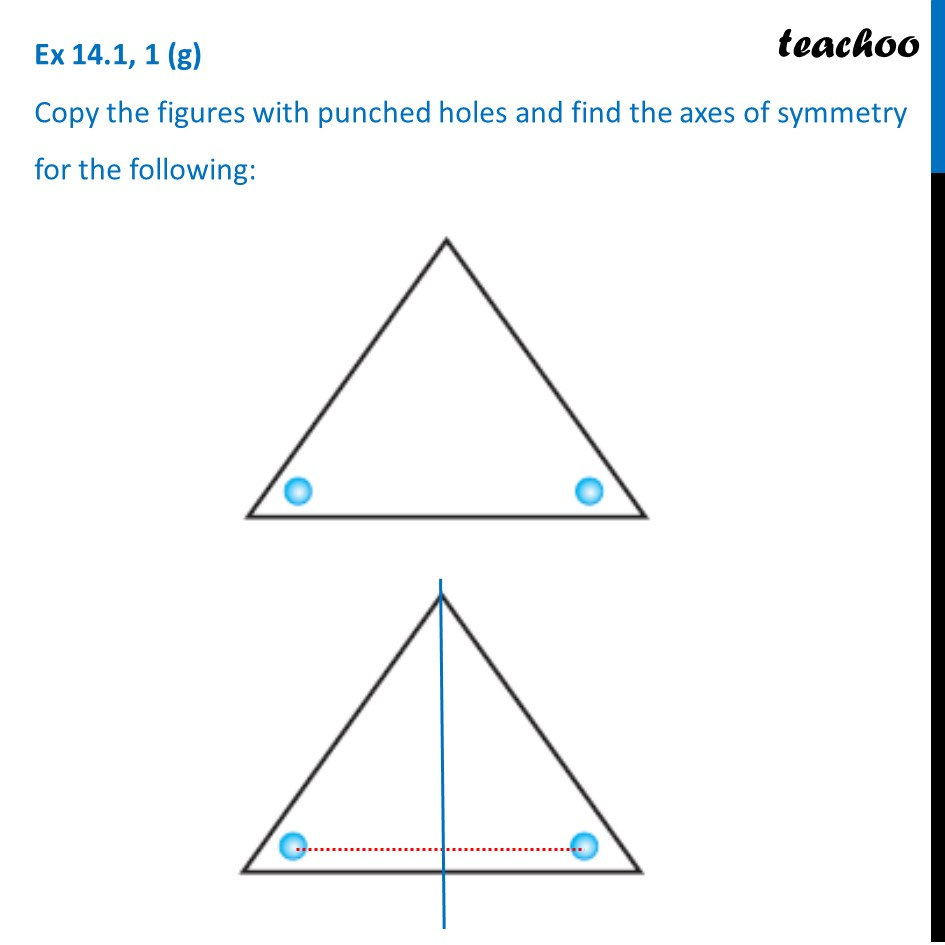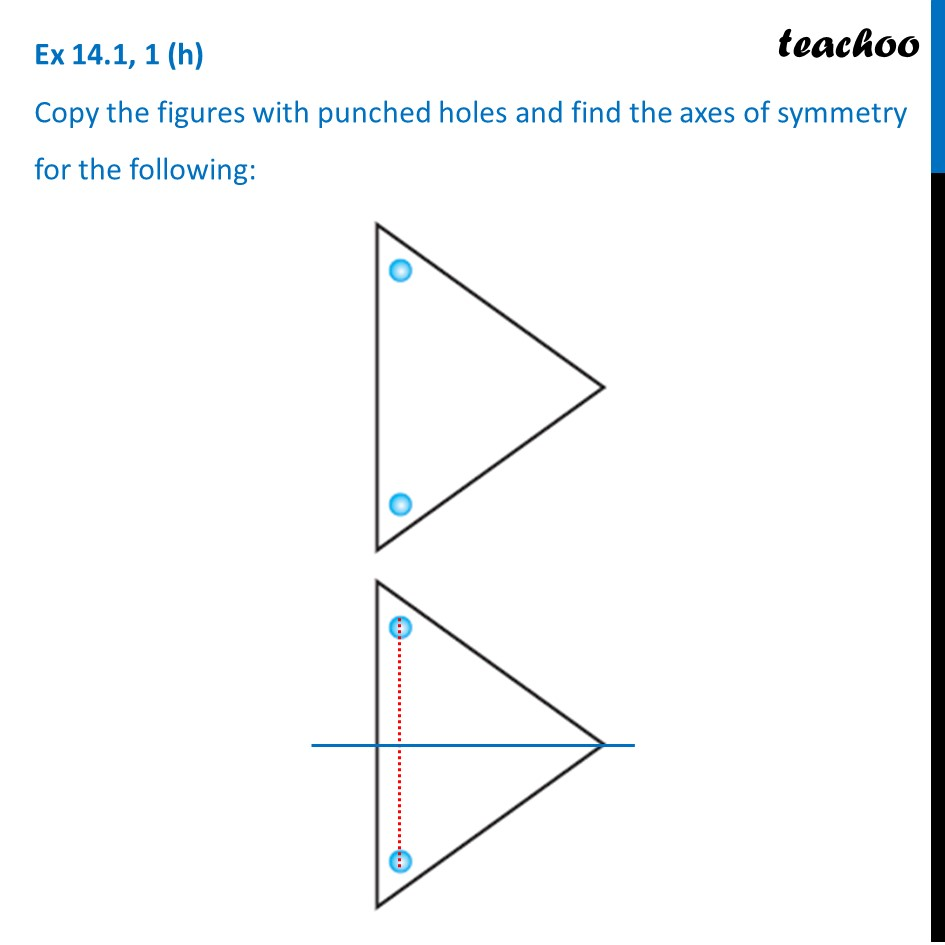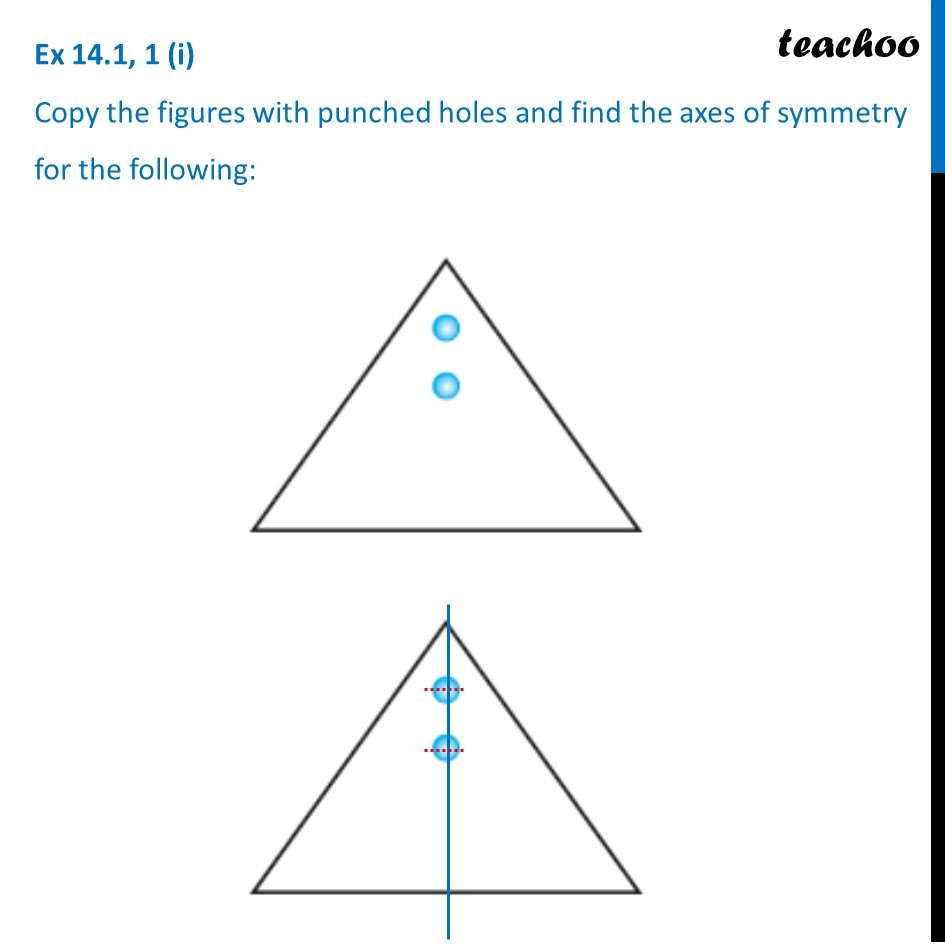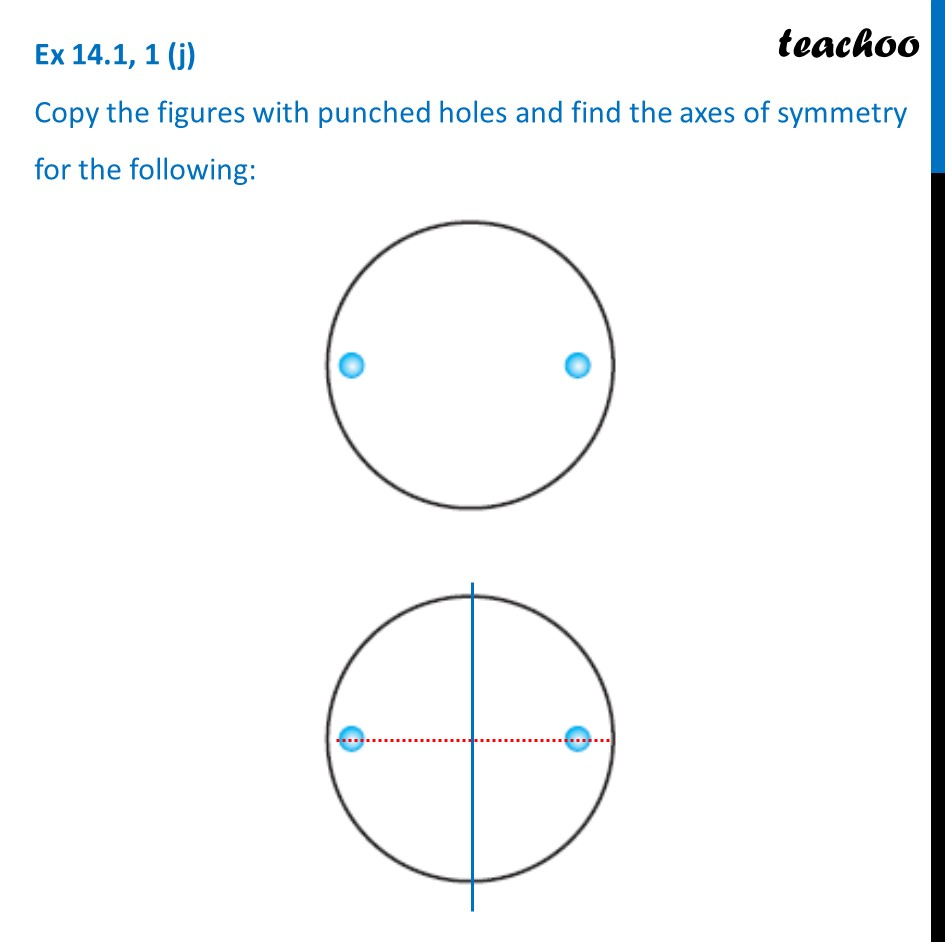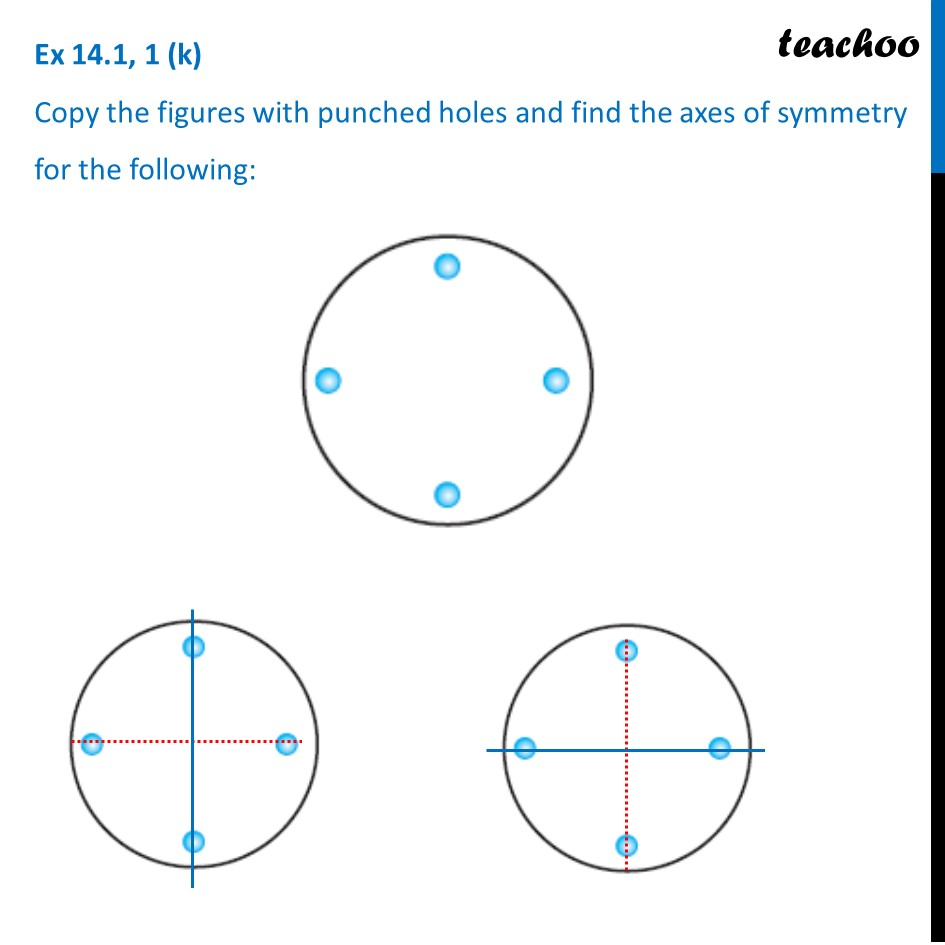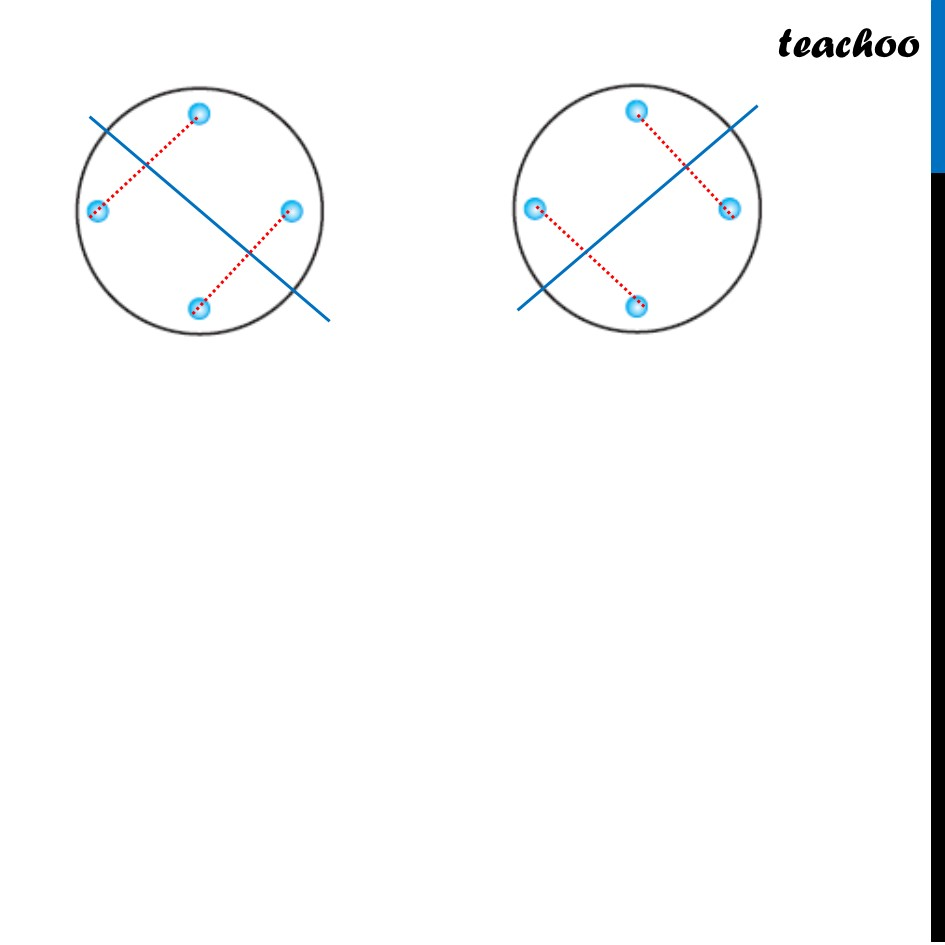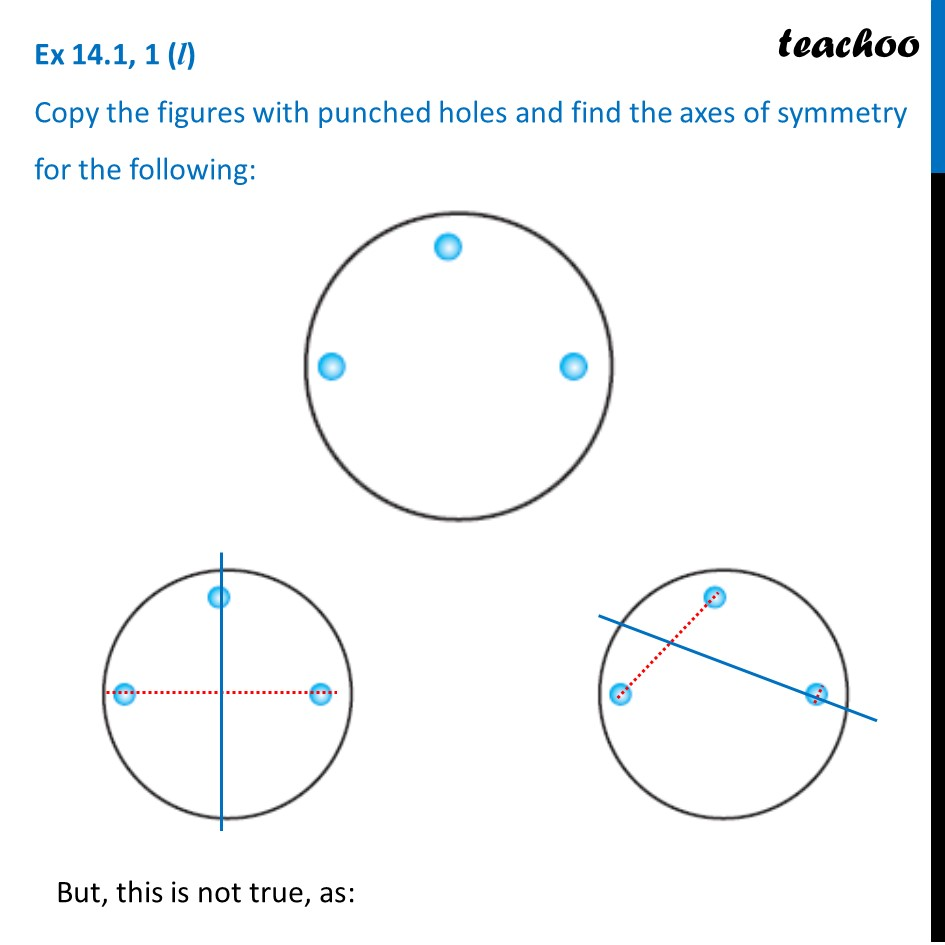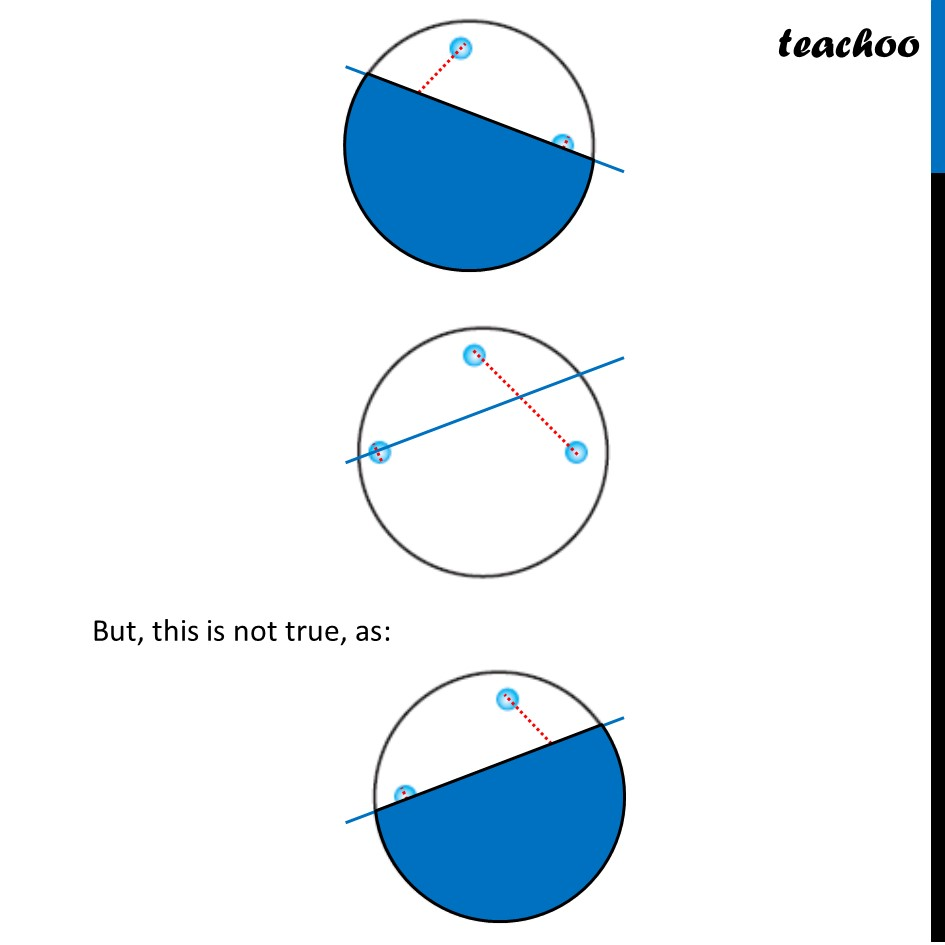Introducing your new favourite teacher - Teachoo Black, at only ₹83 per month

### Transcript

Ex 14.1, 1 (a) Copy the figures with punched holes and find the axes of symmetry for the following: To find line of symmetry, We join points, and draw a line perpendicular to it Then, we check if it is line of symmetry Ex 14.1, 1 (b) Copy the figures with punched holes and find the axes of symmetry for the following: To find line of symmetry, We join points, and draw a line perpendicular to it Then, we check if it is line of symmetry Ex 14.1, 1 (c) Copy the figures with punched holes and find the axes of symmetry for the following: To find line of symmetry, We join points, and draw a line perpendicular to it Then, we check if it is line of symmetry Ex 14.1, 1 (d) Copy the figures with punched holes and find the axes of symmetry for the following: To find line of symmetry, We join points, and draw a line perpendicular to it Then, we check if it is line of symmetry If this would have been line of symmetry, The other whole would be on green spot. Hence, it is not the line of symmetry If this would have been line of symmetry, The other whole would be on green spot. Hence, it is not the line of symmetry Ex 14.1, 1 (e) Copy the figures with punched holes and find the axes of symmetry for the following:Ex 14.1, 1 (f) Copy the figures with punched holes and find the axes of symmetry for the following: But, this is not true, as: But, this is not true, as: Ex 14.1, 1 (g) Copy the figures with punched holes and find the axes of symmetry for the following: Ex 14.1, 1 (h) Copy the figures with punched holes and find the axes of symmetry for the following: Ex 14.1, 1 (i) Copy the figures with punched holes and find the axes of symmetry for the following: Ex 14.1, 1 (j) Copy the figures with punched holes and find the axes of symmetry for the following: Ex 14.1, 1 (k) Copy the figures with punched holes and find the axes of symmetry for the following: Ex 14.1, 1 (l) Copy the figures with punched holes and find the axes of symmetry for the following: But, this is not true, as: But, this is not true, as: